# CBSE Worksheet for Chapter - 1 Surds and Exponents Class 8

## Find CBSE Worksheet for chapter-1 Surds and Exponents class 8

1. CLASS-8
2. BOARD: CBSE
3. Mathematic Worksheet - 1
4. TOPIC: Surds and Exponents
5. For other  CBSE Worksheet for class 8 Mathematic  check out main page of Physics Wallah.

#### SUMMARY:

Let ‘a’ be a rational number and ‘n’ be a positive integer such that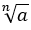is an irrational, thenis called a surd of the order n and a is called the radical.is a surd if a is a rational number and is an irrational number.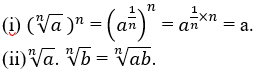#### Exponents:

Law of exponents: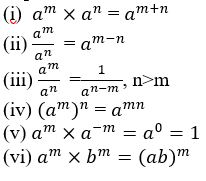#### EXERCISE:

1. If 3x = 500, then the value of 3x-2 is:
1. 100/9
2. 1000/9
3. 500/9
4. 500/3
2. If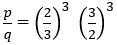= then the value of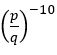is:
1. 1
2. 0
3. cannot be determined
4. None of these
3. The value of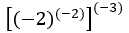is:
1. 64
2. 32
3. Cannot be determined
4. None of these
4. Simplify: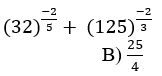1. 4/25
2. 25/4
3. 2/5
4. 5/2
5.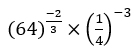equals to:
1. 4
2. 1/4
3. 1
4. 16
6. If x + 1/x = 2, then is equal to:
1. 1
2. 3
3. 2
4.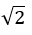7. The value of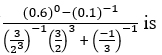is
1.   3/2
2. = 3/2
3. 2/3
4. - 1/2
8. The exponential form of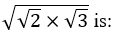is:
1.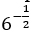2.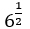3.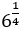4. 6
9. The value of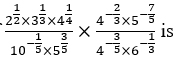1. 10
2. 1
3. 6
4. 8
10. Which of the following is equal to 1?
1. A)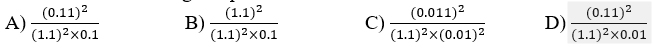11. If apaq=ax, then x=
1. A) p+q
2. B) p-q
3. C) pq
4. D) p/q
12. The value of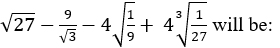will be:
1.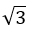2. 23. 0
4. 313. If (4)3 x (6)4 x (10)5 = 2x x 3y x 5z  then the value of x+y+z is:
1. 12
2. 15
3. 20
4. 24
14. If b = a + c, then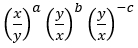=
1. 0
2. 1
3. 2
4. – 1
15. The value of ‘a’ in the following equation: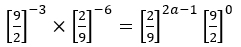1. 1
2. - 2
3. - 1
4. 2

1. (C)
2. (A)
3. (A)
4. (B)
5. (A)
6. (C)
7. (B)
8. (C)
9. (A)
10. (C)
11. (A)
12. (C)
13. (D)
14. (B)
15. (C)